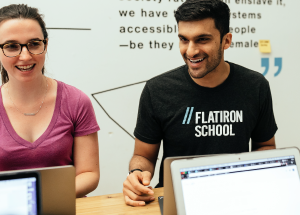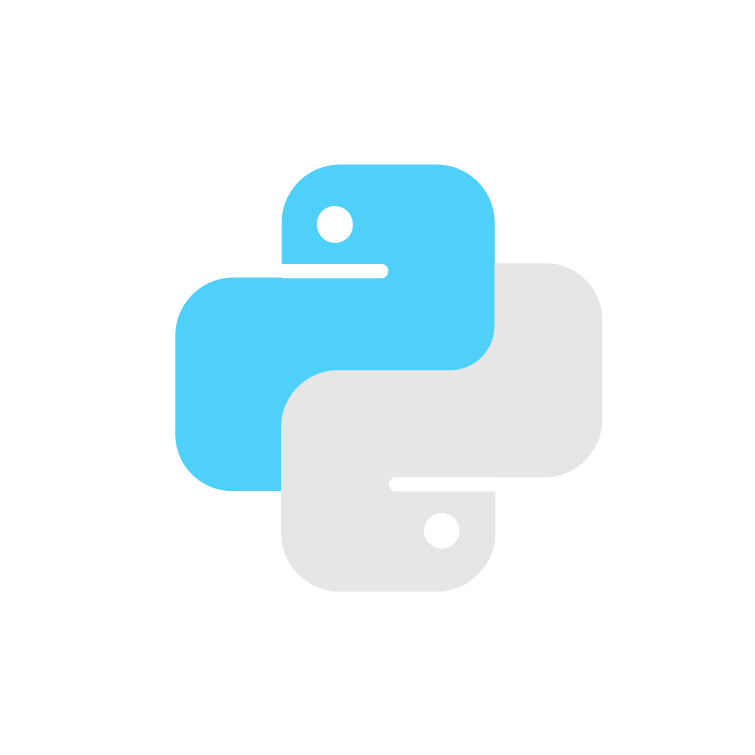# Intro to Binomial Distribution

Every day, hotels juggle their offerings and vacancy to maximize profits. Using this real-world scenario, our free tutorial teaches you about binomial distribution and the Bernoulli experiment.

## Why learn about binomial distribution?Our binomial distribution lessons are free — that way you can set your schedule and learn the fundamentals at your own pace.Our lessons are the best way to experience if learning data science is for you. Explore whether you’re passionate about pursuing a career in data, or if you just want to brush up on some skills.Prepare for a data science bootcamp

Intro to Binomial Distribution is an efficient way to get real hands-on experience with the data analytics fundamentals you’ll need to get into our immersive data science course.

## Our grads work at some of tech's biggest companies## Introduction to binomial distribution: what you'll learn

The travel and hospitality industries have become reliant on technology to help them make and keep their operations profitable. For hotel owners and managers, one thing they work toward is filling as many rooms as possible while getting the best prices.

In these lessons, we will explore one method to help maximize profitability by optimizing hotel booking using Python.Designing a Bernoulli Experiment

Learn how to build a Bernoulli experiment and what the results of an experiment represent.Visualizing a Bernoulli Experiment

Learn how to use a Bernoulli experiment and the probability of customers not showing up for a booked room to calculate the best number of rooms to overbook for maximum profitability.Using Python to Analyze Results

Use matplotlib.pyplot in the Python library to graph your experiment results.

## Other free data science tutorialsIntroduction to Python

Using a list of top travel locations, learn about list data structure and how to manipulate its contents.Intro to Word Clouds & Python

Learn how to analyze famous song lyrics using word clouds, Python, and data visualization.What is binomial distribution?

The binomial distribution describes the probability of a “success” versus “failure” when running an experiment multiple times in a row. A typical application of a binomial process is a coin toss, where you have two possible outcomes: either heads or tails. There are many applications of the binomial distribution, but any process that is defined by the binomial distribution has 2 and only 2 possible outcomes.

When do you use binomial distribution?

When you are running multiple Bernoulli experiments, and you want to get a better sense of what the probability is of observing success (or failure), you can use the binomial distribution.

What is a Bernoulli experiment?

A Bernoulli experiment is an experiment that has two possible outcomes, generally described as “failure” or “success”. A typical example of the Bernoulli experiment is flipping a coin. When flipping a coin, the probability of head is 0.5, as well as the probability of tail, which is the other possible event. Bernoulli outcomes don’t necessarily need to have a split 50-50% chance. For example, scoring a penalty kick in soccer is an example of a Bernoulli experiment, where the chance of scoring is generally higher (~80%) than the chance of missing (~20%).

Is Flatiron School's free binomial distribution tutorial really free?

Yes, our Introduction to Binomial Distribution & Bernoulli Experiments is entirely free , just like the rest of our workshops and tutorials.

If you're looking to learn binomial distribution and understand the Bernoulli experiments, this is a perfect place for you to start.

What courses and workshops does Flatiron School offer?

Flatiron School teaches students the skills they need to become a software engineer, data scientist, cybersecurity engineer or analyst, or product designer.

If you're looking to start a new career, those are the courses for you.

We also offer free introductory experiences for beginners in coding, data science, and cybersecurity.

Coding

Data Science

Cybersecurity